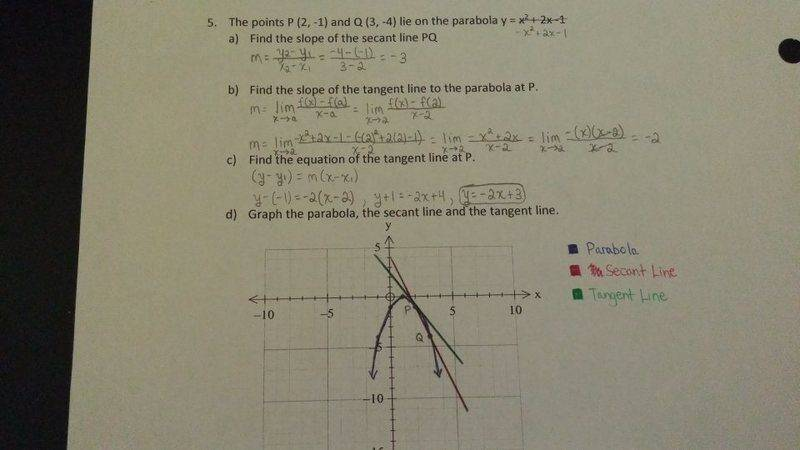# Finding the Slope of Secant and Tangent Lines

cvc121

## Homework Statement

The points P (2,-1) and Q (3,-4) lie on the parabola y = -x2+2x-1
a) Find the slope of the secant line PQ.
b) Find the slope of the tangent line to the parabola to the parabola at P.
c) Find the equation of the tangent line at P.

## The Attempt at a Solution

I am just new to calculus and am having trouble determining the slope of a secant and tangent line. My attempt at the questions are attached. Can anyone verify my work to see if I am on the right track? Are my methods for solving for the slopes correct? Thanks! All help is very much appreciated!

#### Attachments

•20160226_203054.jpg
40.7 KB · Views: 696

brainpushups
Yup. Looks good.

Staff Emeritus
Homework Helper
Gold Member

## Homework Statement

The points P (2,-1) and Q (3,-4) lie on the parabola y = -x2+2x-1
a) Find the slope of the secant line PQ.
b) Find the slope of the tangent line to the parabola to the parabola at P.
c) Find the equation of the tangent line at P.

## The Attempt at a Solution

I am just new to calculus and am having trouble determining the slope of a secant and tangent line. My attempt at the questions are attached. Can anyone verify my work to see if I am on the right track? Are my methods for solving for the slopes correct? Thanks! All help is very much appreciated!
It looks fine.